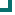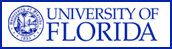Enterococcus faecalis (NORTHEAST Data) 2004 2005 2006 2007 2008 2009 2010 penicillin 97.8%n=3345 97%n=3068 97%n=4997 96.7%n=4141 97.8%n=4382 97.4%n=1495 ampicillin 98.5%n=1987 96.7%n=1379 98.3%n=3910 97.8%n=4000 98.1%n=4478 98.4%n=1486 vancomycin 96.8%n=3310 97.5%n=2953 95.1%n=4891 95%n=4795 96.3%n=4911 95.7%n=1784 quinupristin/dalfopristin 3.9%n=611 4%n=630 3.1%n=708 0%n=160 0%n=167 ciprofloxacin 49.5%n=1713 46%n=346 49.7%n=1584 49.9%n=1146 49.3%n=1343 62.4%n=862 levofloxacin 54.3%n=2126 40%n=1979 51.9%n=1506 51.9%n=2103 53.7%n=1543 66%n=520 nitrofurantoin 99.3%n=1765 99.9%n=2108 96%n=3834 94.8%n=3302 98.8%n=3605 98.9%n=1059 tetracycline 29.5%n=2550 34.3%n=1722 28.9%n=3338 26.2%n=3509 24.8%n=3679 23.9%n=862 gentamicin 68.6%n=973 69.5%n=586 71.9%n=800 67.9%n=442 4.9%n=431 tobramycin 30%n=267 amikacin 100%n=50 chloramphenicol 89.7%n=1092 93.1%n=319 89.2%n=1459 85.9%n=369 rifampim 0%n=14 Totals 75%n=19472 75.8%n=15357 79.4%n=27027 78.3%n=23598 79.1%n=24922 83.9%n=8118

This data is accurate as of: 11/22/2016 11:53:43 AM

Drugs with less than 10 isolates are removed from the chart and from the graph. The following drugs had some data removed: rifampim, amikacin.ARMProgram.com is a project run by the University of Florida. Copyright 2011. All Rights Reserved. Site by JMarc Technologies, LLC